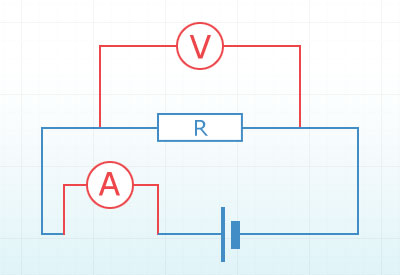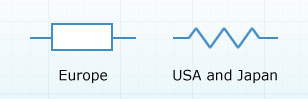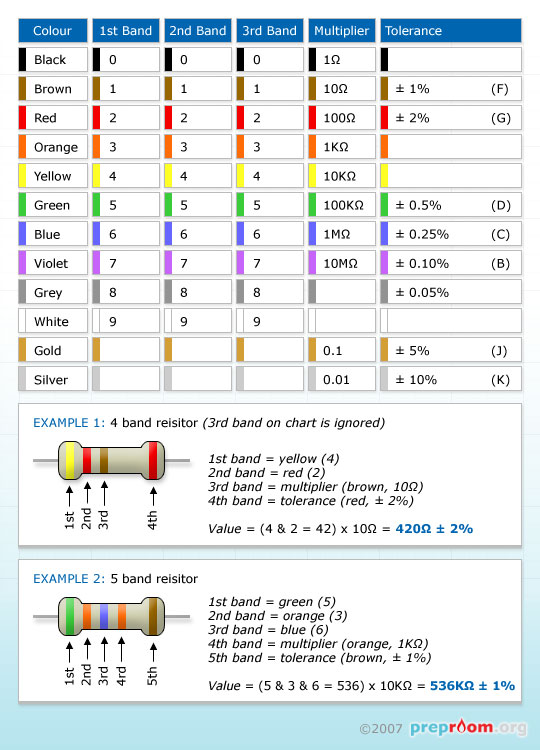You are here: Info Library > Electricity > Resistors
Info Library
Options

# Electricity > Resistors

A resistor is an electrical component with two terminals which when in a circuit, resists an electric current. The amount it resists depends upon its value.

The measure of electrical resistance is equal to the voltage drop across the resistor divided by the current flowing through the resistor. This equation is known as Ohm's Law which is expressed as:

R = V/I

where R= resistance, V= voltage and I= current.

To simplify things slightly and to make sense of those letters, take a look at the diagram below. It shows a simple resistor circuit containing an ammeter and voltmeter. Voltmeters should always be connected across the resistor as they are there to measure the voltage between the resistor's terminals. The ammeter measures the current that flows around the circuit and through the resistor.

Now let's say the resistor is of an unknown value (ie we don't know how many ohms it is) but we put it into this circuit and the ammeter reads 1A and the voltmeter reads 6V. With these results we can easily work out the resistance of the resistor using Ohm's Law.

We know the 'V' and we know the 'I' so to work out the 'R' we just put those figures into the equation like this: R=6/1 (resistance = 6 divided by 1) which gives us a resistor value of 6Ω.Fig 1:
Simple resistor circuit

We can rearrange the Ohm's Law equation to work out the Voltage (if we know the resistance and current) or the Current (if we know the voltage and resistance) By using the Ohm's Law triangle below, we can easily rearrange this equation.

If you place your thumb over the unknown letter, for example the R, you are left with the V over I and therefore R=V/I. Using the triangle method will help you to rearrange other three way equations.Fig 2:
Ohm's Law Triangle

The SI unit of resistance is the ohm. (symbol: Ω) and its symbol used in electrical circuit diagrams is below. In Europe we use an empty rectangle which may include the resistor value in ohms. In America and Japan they use a zigzag. You may see this symbol in some older textbooks and is prevalent on the internet so is worth knowing they mean the same thing.Fig 3:
Resistor symbols

Values range from single ohms to millions of ohms. Letters and numbers are used to denote these variations which will appear in textbooks and exam papers. For example:

1R = 1 ohm (R means ohm)
1kΩ = 1 kilo-ohm or 1000 ohms
1MΩ = 1 mega-ohm

Just to complicate matters, numbers appearing before the letter indicate a value more that one, letters coming after the letter indicate a value less that one. For example:

50R = 50Ω and R50= 0.50Ω
2M = 2MΩ
and 2M2 = 2.2MΩ

Most resistors have coloured banding which is used to identify its value in ohms. Four-band identification is the most common and involves coloured bands that are painted around the resistor body.

The first band represents tens and the second band units. Each colour stands for a different unit (see table below) The third band is a multiplier and the forth a 'tolerance' band. This last band shows how accurate the resistor is. Because manufacturers cannot guarantee the exact resistance acquired from the first three bands the forth shows what percentage of accuracy the resistor has.

The table below can be used to determine the values of four and five band resistors. When valuing a four band resistor simply ignore the third band column.Fig 4:
Resistor bands

In the two examples above, the top four band resistor (example 1) has a value of 420Ω plus or minus 2% and the bottom (example 2) five band resistor has a value of 536KΩ plus or minus 1%.